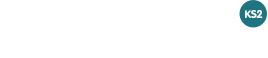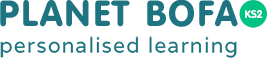#THE PLANET BOFA DIFFERENCE

# KS2 Maths Arithmetic Syllabus

Here you can find example questions covering the KS2 Maths Arithmetic Syllabus. You can also try a demo test to get a better understanding of how BOFA works. The syllabus has been taken from the National Curriculum.

Component Strand Sub-strand Year 3 NC Ref / Description Year 4 NC Ref / Description Year 5 NC Ref / Description Year 6 NC Ref / Description
Number Calculations: Addition, subtraction, multiplication and division Add / subtract using written methods
Number Calculations: Addition, subtraction, multiplication and division Multiply / divide using written methods
Number Calculations: Addition, subtraction, multiplication and division Multiply / divide using written methods
Number Calculations: Addition, subtraction, multiplication and division Order of operations
Number Fractions, decimals and percentages Add / subtract fractions
Number Fractions, decimals and percentages Multiply / divide fractions
Number Fractions, decimals and percentages Multiply / divide fractions
Number Fractions, decimals and percentages Multiply / divide decimals
Number Fractions, decimals and percentages Multiply / divide decimals
Number Fractions, decimals and percentages Add / subtract decimals
Number Ratio and proportion Percentage of quantities